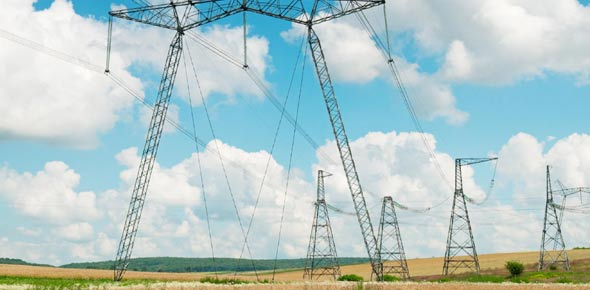# Electricity Basic Principles And Applications

10 Questions | Total Attempts: 336SettingsBasic Electricity Questions and Concepts

• 1.
The ability to do work is a definition of
• A.

Charge

• B.

Energy

• C.

Neither of the above

• D.

Both of the above

• 2.
The joule is the base unit of
• A.

Energy

• B.

Work

• C.

Neither of the above

• D.

Both of the above

• 3.
A negative ion has an excess of
• A.

Electrons

• B.

Protons

• C.

Neither of the above

• D.

Both of the above

• 4.
The nucleus of the most atoms contain
• A.

Neutrons

• B.

Protons

• C.

Neither of the above

• D.

Both of the above

• 5.
All matter is made up of only
• A.

Neutrons

• B.

Nucleuses

• C.

Neither of the above

• D.

Both of the above

• 6.
A positive charge can
• A.

Attract a negatively charged object

• B.

Attract and then repel a neutral object

• C.

Neither of the above

• D.

Both of the above

• 7.
The part of the atom that has no electric charge is the
• A.

Neutron

• B.

Nucleus

• C.

Neither of the above

• D.

Both of the above

• 8.
A proton has
• A.

A positive electric charge

• B.

A negative electric charge

• C.

Neither of the above

• D.

Both of the above

• 9.
Electrons in the outermost shell of an atom are called
• A.

Free electrons

• B.

Valence electrons

• C.

Neither of the above

• D.

Both of the above

• 10.
When an electron becomes a free electron its energy level is
• A.

Cut in half

• B.

Unchanged

• C.

Double

• D.

None of the above

Related TopicsBack to top